# A dynamical computation of a multiple integral involved in the neutron star theory

This study consists in computing dynamically using the CADNA library an integral involved in the neutron star theory. Massive stars collapse with an explosion ejecting all the matter, except a central residue: a neutron star or a black hole, depending on the details of the scenario. Neutron stars are mainly done of neutrons with a typical proportion of protons smaller than 10%. With time, equilibrium between protons and neutrons takes place. Time to reach this equilibrium is obtained by calculating high order integrals involving several variables for any particle appearing in the reactions.

The integral, which has been provided by Loïc Villain (LUTH, Observatoire de Paris-Meudon, France), is the following: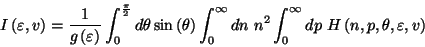whereandis a normalization function.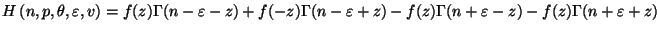,

withand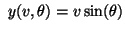.

To compute, several numerical problems have to be faced:

• The integral has two infinite bounds. Each integral of the form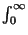is replaced by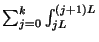. One problem is the choice of an optimal value for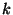.
•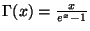generates cancellations if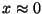.
Therefore a series expansion of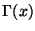is used: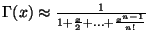One problem is the choice of an optimal value for.
•is the composition of three one-dimensional integrals. A quadrature method, such as Gauss-Legendre method, is used to compute each one-dimensional integral. The truncation error generated by this quadrature method is another problem.is expressed as one-dimensional integrals: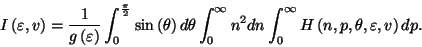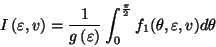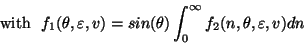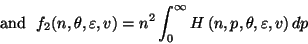Each one-dimensional integral is evaluated by two iterative processes:

• A sequence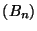of integrals on a finite interval with an increasing upper bound:The sequenceis computed until the difference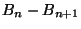is not significant.
• Each integral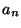is computed using Gauss-Legendre method.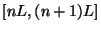is partitioned insub-intervals of length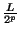. The integral on each sub-interval is approximated using the classical Gauss-Legendre method. Let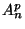be the approximation ofusing Gauss-Legendre method onsub-intervals of. The sequence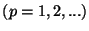is computed until the differenceis not significant.

Using this strategy, the exact singificant digits of the approximation ofobtained are those of the exact value of the integral, up to 6.

To get the FORTRAN source code.